Win up to 100% scholarship on Aakash BYJU'S JEE/NEET courses with ABNAT Win up to 100% scholarship on Aakash BYJU'S JEE/NEET courses with ABNAT

# Chemical Bonding – JEE Advanced Previous Year Questions with Solutions

Chemical Bonding JEE Advanced Previous Year Questions with Solutions are provided here to make studying an easy and interesting process. Students will find all the important previous year questions for the Chemical Bonding chapter in JEE Advanced syllabus. We have also provided the solutions which will further serve as excellent study material that will help JEE aspirants to develop the right approach to tackle different types of questions.

Chemical Bonding JEE Advanced Previous Year Questions with Solutions are aimed at helping students solve even the most complicated problems with speed and accuracy. Students can download the questions and solutions as free PDf downloads. More significantly, students will be greatly benefited and they will understand how easy it is to analyze the questions and score high marks in the entrance exam.

## JEE Advanced Previous Year Questions on Chemical Bonding

Question 1. Consider the following compounds in the liquid form:

O2, HF, H2O, NH3, H2O2, CCl4, CHCl3, C6H6, C6H5Cl

When a charged comb is brought near their flowing stream, how many of them show deflection as per the following figure?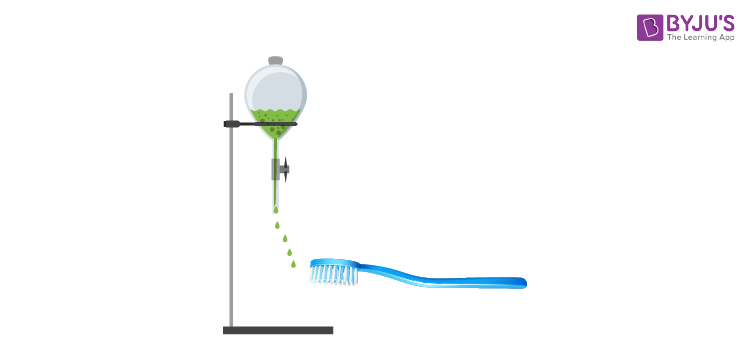Solution: (6)

Only polar molecules are deflected by charged comb.

Polar molecules: HF, H2O, NH3, H2O2, CHCl3, C6H5Cl

Non-polar molecules: O2, CCl4, Benzene

Therefore, when a charged comb is brought near their flowing stream, six compounds will show deflection.

Question 2. Among B2H6, B3N3H6, N2O, N2O4, H2S2O3 and H2S2O8, the total number of molecules containing covalent bond between two atoms of the same kind is_____.

Solution: (4)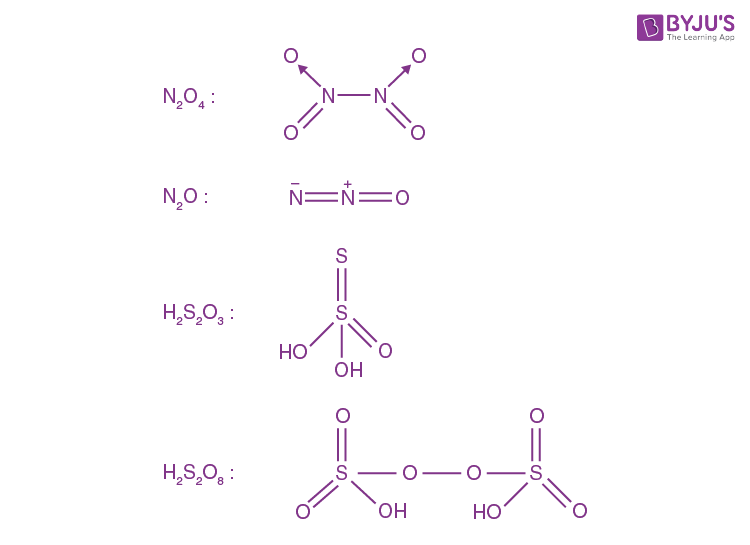∴ Number of compounds having bond between the same type of atoms = 4

Question 3. Each of the following options contains a set of four molecules. Identify the option(s) where all four molecules possess permanent dipole moment at room temperature.

A. NO2, NH3, POCl3, CH3Cl

B. BF3, O3, SF6, XeF6

C. BeCl2, CO2, BCl3, CHCl3

D. SO2, C6H5Cl, H2Se, BrF5

Solution: (A, D)

If two identical dipole vectors are oriented at an angle of 180o to each other, they cancel each other resulting in zero net dipole moment. Example. CO2 and BeCl2

If three identical dipole vectors are oriented at an angle of 120o, they cancel each other out resulting in zero net dipole moment. eg BF3

SF6 also has a perfectly symmetrical octahedral geometry. Hence it also does not possess a permanent dipole moment at room temperature.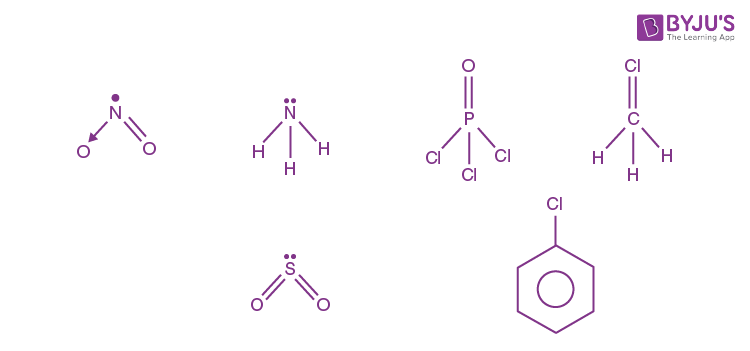Question 4. The sum of the number of lone pairs of electrons on each central atom in the following species is.

[TeBr6]2-, [BrF2]+, SNF3 and [XeF3]

(Atomic numbers: N = 7, F = 9, S = 16, Br = 35, Te = 52, Xe = 54)

Solutions: (6)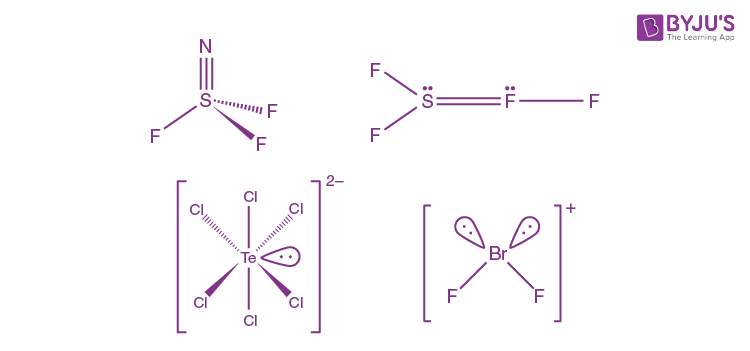From the diagrams we can say that;

Te atom in [TeBr6]2- has 1 lone pair

Br atom in [BrF2]+ has 2 lone pairs

S atom in SNF3 has 0 lone pair

Xe atom in [XeF3] has 3 lone pairs

Therefore, the sum of the number of lone pairs of electrons on each central atom in the given species = 1 + 2 + 0 + 3 = 6.

Question 5. Among the triatomic molecules/ions, Be Cl2, N3-, N2O, NO+2, O3, SCl2, ICl2, l3 and XeF2, and XeF2, the total number of linear molecules(s)/ion(s) where the hybridization of the central atom does not have contribution from the d-orbital(s) is [Atomic number:S = 16, Cl = 17, I = 53 and Xe = 54]

Solution: (4)

The total number of the linear molecule(s)/ion(s) where the hybridization of the central atom does not have a contribution from the d-orbital(s) is 4.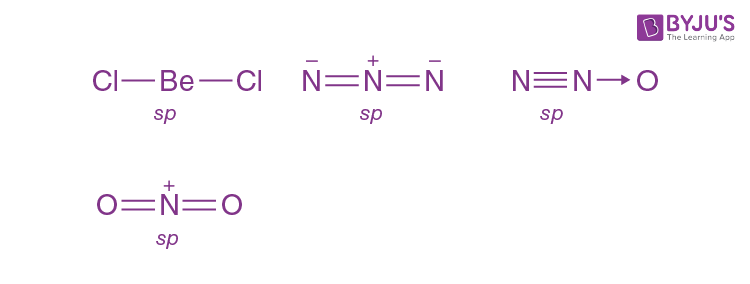They are BeCl2, N3, N2O, NO2+.

In the remaining molecules/ions, O3 is bent, SCl2 is V-shaped with sp3 hybridization, ICl2, I3 and XeF2, are linear with sp3d hybridization.

Question 6. A list of species having the formula XZ4 is given below:

XeF4, SF4, SiF4, BF4, BrF4, [Cu(NH3)4]2+, [FeCl4]2-, CoCl42- and [PtCl4]2-

Defining shape on the basis of the location of X and Z atoms, the total number of species having a square planar shape is.

Solution: (4)

XeF4 = Square planar

SF4 = See-saw

SiF4 = Tetrahedral

BF4 = Tetrahedral

BrF4 = Square planar

[Cu(NH3)4]2+ = Squara planar

[FeCl4]2- = Tetrahedral

CoCl42- = Tetrahedral

[PtCl4]2-= Squara planar

Question 7. Based on VSEPR theory, the number of 90 degrees F-Br-F angles in BrF5, is.

A. 8

B. 4

C. 0

D. 6

Solution: (C)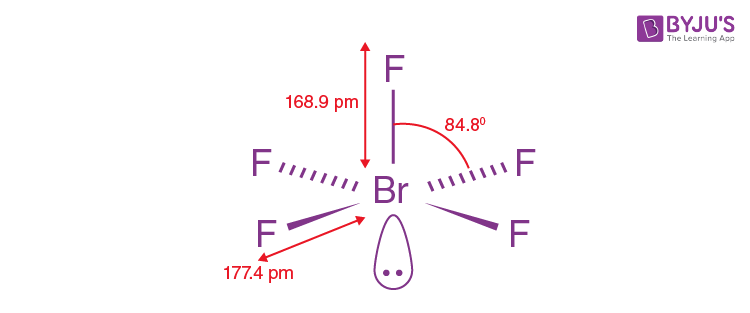In BrF5 there are 5 bond pairs and 1 lone pair on Br atom and hence the hybridization is sp3d2 geometry and shape are octahedral and square pyramidal respectively. Due to lp-bp repulsions, the bond angles are lower than 90o (as in octahedral). Hence there are no bond angles with 90o.

Question 8. The compound (s) with two lone pairs of electrons on the central atom is (are)

A. BrF5

B. CIF3

C. XeF4

D. Sf4

Solution: (B, C)

Only, CIF3 and XeF4 contain two pairs of electrons on the central atom.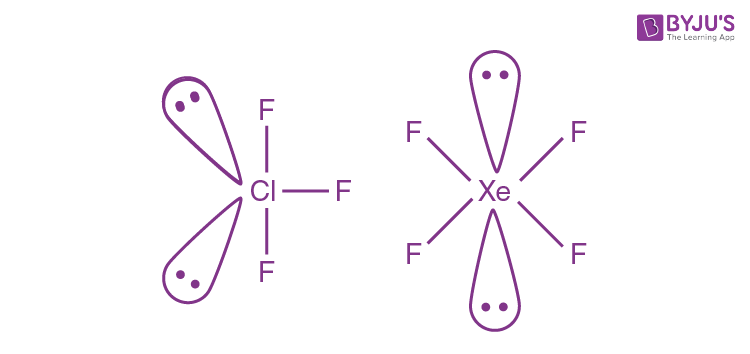Question 9. Assuming 2s – 2p mixing is not operative the paramagnetic species among the following is:

A. Be2

B. B2

C. C2

D. N2

Solution: (C)

If 2 s − 2 p 2s-2p mixing is not operative , then molecular orbitals may be arranged in order of energy as follows:

σ(1s), σ*(1s), σ(2s), σ*(2s), σ(2pz), π(2px) = (2py), π*(2px) = π*(2py), σ*(2pz)

Applying this configuration, BE2, B2 and N2 will be diamagnetic but C2 will be paramagnetic.

Question 10. Among H2, He2+, Li2, Be2, B2, C2, N2,O2 and F2, the number of diamagnetic species is (Atomic numbers: H = 1, He = 2, Li = 3, Be = 4, B = 5, C = 6, N = 7, O = 8, F = 9)

Solution: (6)

Molecular orbitals configurations: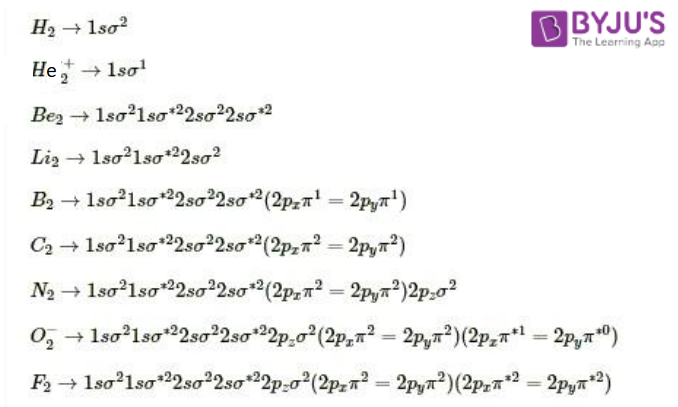Diamagnetic species → All electrons paired

H2, Li2, Be2, C2, N2 and F2 are diamagnetic species.

However, the bond order of Be2 is zero thus Be2 does not exist. So, the number of diamagnetic species is 6.